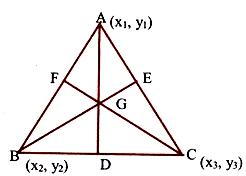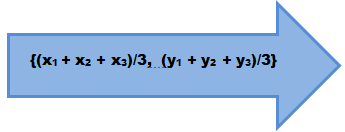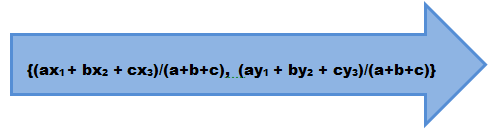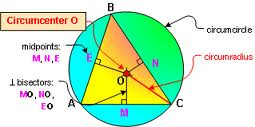×#### Thank you for registering.

One of our academic counsellors will contact you within 1 working day.

Click to Chat

1800-1023-196

+91-120-4616500

CART 0

• 0

MY CART (5)

Use Coupon: CART20 and get 20% off on all online Study Material

ITEM
DETAILS
MRP
DISCOUNT
FINAL PRICE
Total Price: Rs.

There are no items in this cart.
Continue Shopping```Centroid, Incentre, Circumcentre and Ex-centre of Triangle

Table of Content

What do you mean by the Centroid of a Triangle?

What do you mean by the Incentre of a Triangle?

What do we mean by the Circumcentre of a Triangle?

What do you mean by Excentre of a Triangle?

What do you mean by Orthocentre of a Triangle?

Related Resources

What do you mean by the Centroid of a Triangle?In order to understand the term centroid, we first need to know what do we mean by a median. A median is the line joining the mid-points of the sides and the opposite vertices. A centroid is the point of intersection of the medians of the triangle. A centroid divides the median in the ratio 2:1.Result:

Find the centroid of the triangle the coordinates of whose vertices are given by A(x1, y1), B(x2, y2) and C(x3, y3) respectively.

Solution:

A centroid divides the median in the ratio 2:1. Hence, since ‘G’ is the median so AG/AD = 2/1.

Since D is the midpoint of BC, coordinates of D are

((x2 + x3)/2, (y2 + y3)/2)

Using the section formula, the coordinates of G are

(2(x2+x3)/2) +1.x1/2+1, (2(y2+y3)/2) +1.y1/2+1)

⇒ Coordinates of G are (x1+x2+x3/3, y1+y2+y3/3).

If the coordinates of a triangle are (x1, y1), (x2, y2) and (x3, y3), then the coordinates of the centroid (which is generally denoted by G) are given byWhat do you mean by the incentre of a triangle?

The incentre of a triangle is the point of intersection of the angle bisectors of angles of the triangle. An incentre is also the centre of the circle touching all the sides of the triangle.

Note:

Angle bisector divides the oppsoite sides in the ratio of remaining sides i.e. BD/DC = AB/AC = c/b.

Incentre divides the angle bisectors in the ratio (b+c):a, (c+a):b and (a+b):c.

Result:

Find the incentre of the triangle the coordinates of whose vertices are given by A(x1, y1), B(x2, y2), C(x3, y3).Solution:

By geometry, we know that BD/DC = AB/AC (since AD bisects ÐA).

If the lengths of the sides AB, BC and AC are c, a and b respectively, then BD/DC = AB/AC = c/b.

Coordinates of D are (bx2+cx3/b+c, by2+cy3/b+c)

IB bisects DB. Hence ID/IA = BD/BA = (ac/b+c)/c = a/c+b.

Let the coordinates of I be (x, y).

Then x = ax1+bx2+cx3/a+b+c, y = ay1+by2+cy3/a+b+c.What do we mean by the Circumcentre of a Triangle?

The point of intersection of perpendicualr bisectors of the sides of a triangle is called the circumcentre of triangle. This is also the centre of the circle, passing through the vertices of the given triangle.The coordinates of circumcentre are given by

{(x1 sin 2A + x2 sin 2B + x3 sin 2C)/ (sin 2A + sin 2B + sin 2C), (y1 sin 2A + y2 sin 2B + y3 sin 2C)/ (sin 2A + sin 2B + sin 2C)}.

What do you mean by Excentre of a Triangle?

Excentre of a triangle is the point of concurrency of bisectors of two exterior and third interior angle. Hence there are three excentres I1, I2 and I3 opposite to three vertices of a triangle.

If A(x1, y1), B(x2, y2) and C(x3, y3) are the vertices of a triangle ABC,Coordinates of centre of ex-circle opposite to vertex A are given as

I1(x, y) = (–ax1+bx2+cx3/a+b+c/–a+b+c, –ay1+by2+cy3/–a+b+c).

Similarly co-ordinates of centre of I2(x, y) and I3(x, y) are

I2(x, y) = (ax1–bx2+cx3/a–b+c, ay1–by2+cy3/a–b+c)

I3(x, y) = (ax1+bx2–cx3/a+b–c, ay1+by2–cy3/a+b–c)

The coordinates of the excentre are given by

I1 = (-ax1 + bx2 + cx3)/(-a + b + c), (-ay1 + by2 + cy3)/(-a + b + c)}

Similarly, we have I2 = (ax1 - bx2 + cx3)/(a - b + c), (ay1 - by2 + cy3)/(a - b + c)}

I3 = (ax1 + bx2 - cx3)/(a + b - c), (ay1 + by2 - cy3)/(a + b - c)}

What do you mean by Orthocentre of a Triangle?

This is the point of concurrency of the altitudes of the triangle.

Key Points:

In a right angled triangle, orthocentre is the point where right angle is formed.

In an isosceles triangle, all of centroid, orthocentre, incentre and circumcentre lie on the same line.

Orthocentre, centroid and circumcentre are always collinear and centroid divides the line joining orthocentre and circumcentre in the ratio 2:1.

Illustration:

If (0, 1), (1, 1) and (1, 0) are middle points of the sides of a triangle, find its incentre.

Solution:

Let A(x1, y1), B(x2, y2) and C(x3, y3)be teh vertices of a triangle.

x1 + x2 = 0, x2 + x3 = 0, x3 + x1 = 0

y1 + y2 = 0, y2 + y3 = 0, y3 + y1 = 0.

Solving these equations, we get A(0, 0), B(0, 2) and C(2, 0).

Now, a = BC = 2√ 2, b = CA = 2 and c = AB = 2.

Thus, incentre of the triangle ABC is (2-√ 2, 2-√ 2).

Related Resources

For getting an idea of the type of questions asked, refer the previous year papers.

To read more, Buy study materials of Straight Lines comprising study notes, revision notes, video lectures, previous year solved questions etc. Also browse for more study materials on Mathematics here.
```### Course Features

• 731 Video Lectures
• Revision Notes
• Previous Year Papers
• Mind Map
• Study Planner
• NCERT Solutions
• Discussion Forum
• Test paper with Video Solution